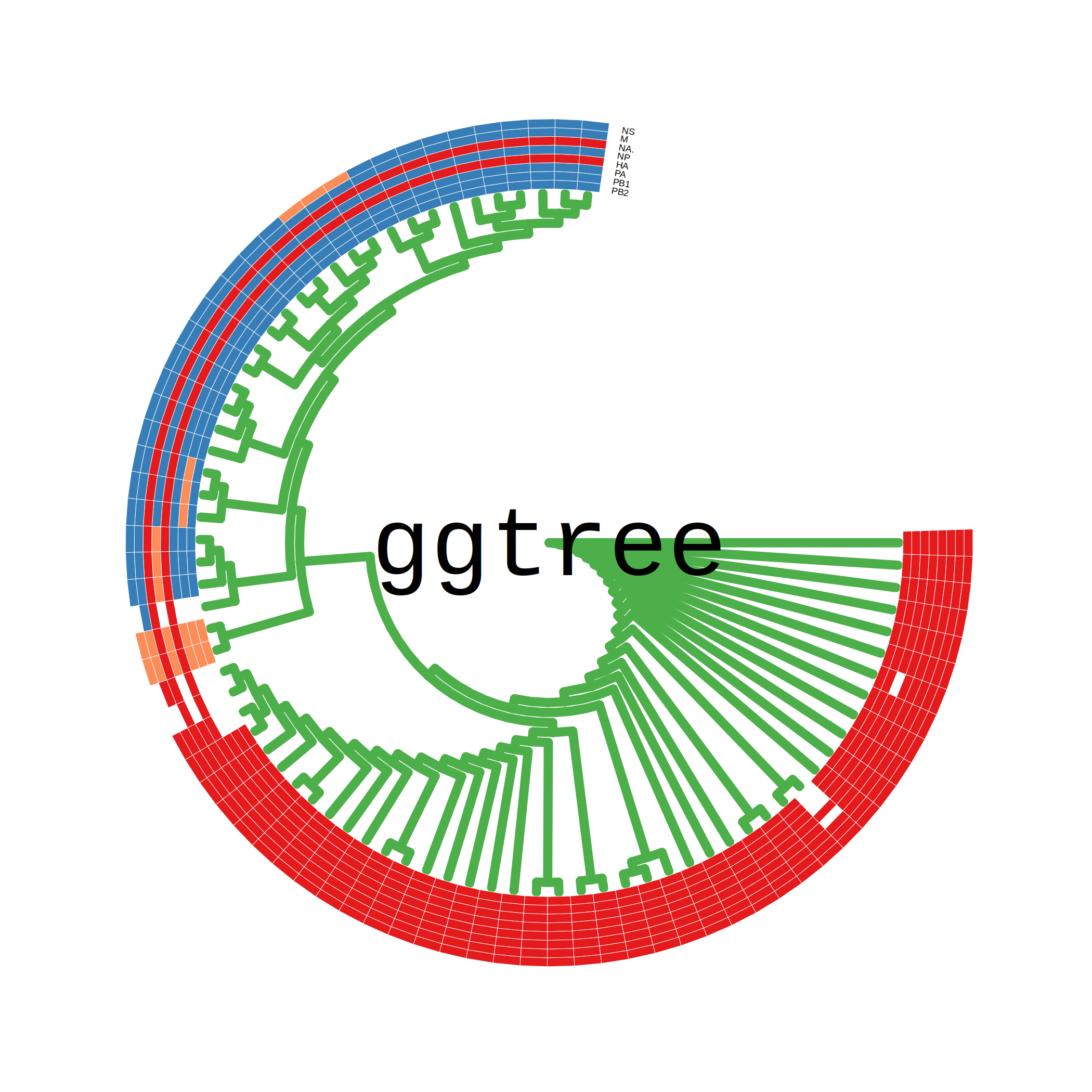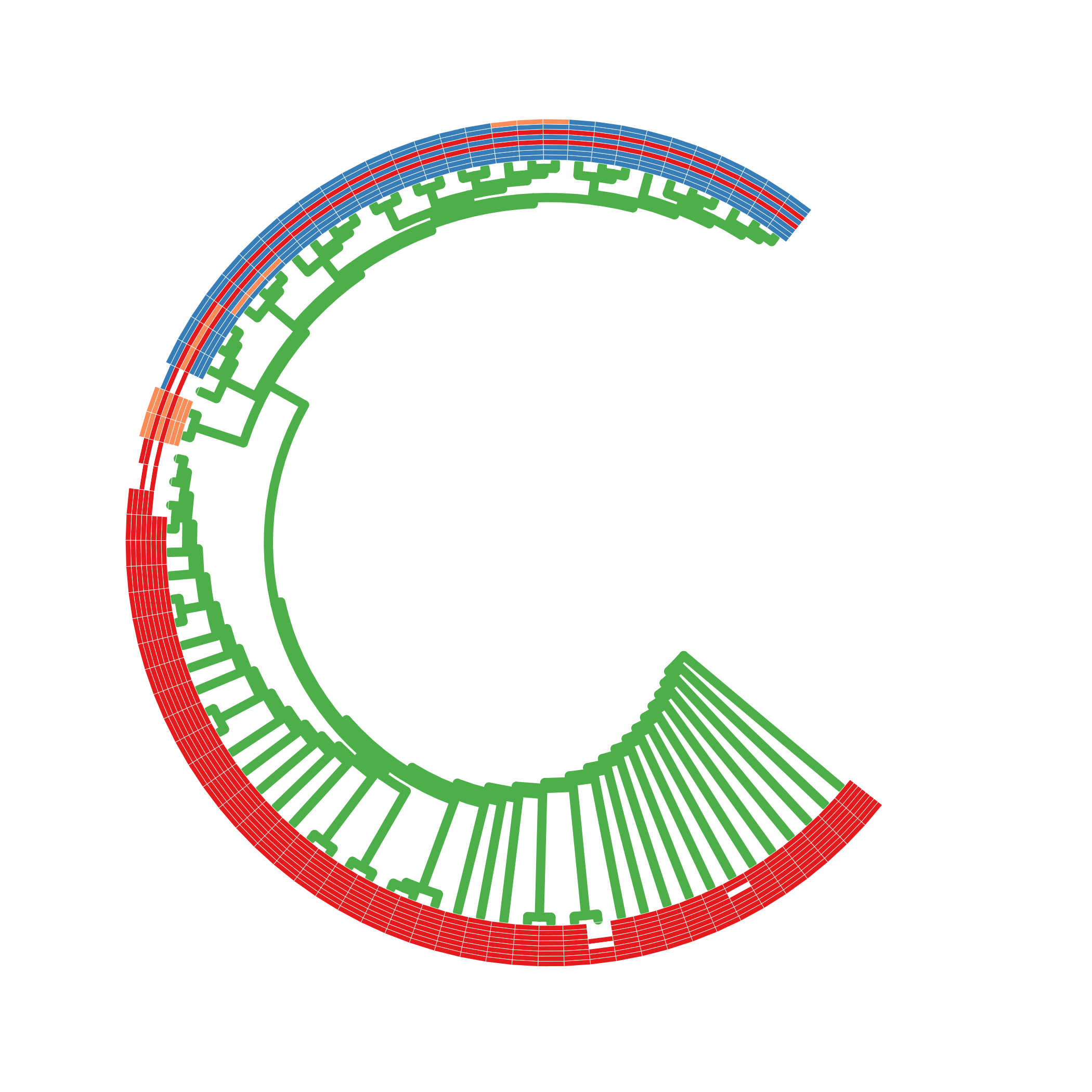ggtree provides many helper functions for manupulating phylogenetic trees and make it easy to explore tree structure visually.

Here, as examples, I used `ggtree` to draw capital character `G` and `C`, which are first letter of my name :-).

To draw a tree in such shape, we need `fan` layout (`circular` layout with open angle) and then rotating the tree to let the open space on the correct position. Here are the source codes to produce the `G` and `C` shapes of tree. I am thinking about using the `G` shaped tree as `ggtree` logo. Have fun with `ggtree` :-)

library(ggtree)

``````beast_file <- system.file("examples/MCC_FluA_H3.tree", package="ggtree")

genotype_file <- system.file("examples/Genotype.txt", package="ggtree")
p <- ggtree(beast_tree, layout='circular', color="#4DAF4A", size=2, branch.length='none', right=T) +
annotate('text', x=0, y=40, label='ggtree', family='mono', size=16)

p2 <- gheatmap(p, genotype, width=0.2, hjust='left', colnames_angle=-10, font.size=1.5)  +
scale_fill_manual(values=c("#E41A1C","#377EB8","#FC8D59")) + theme_tree()

open_tree(p2, 80) %>% rotate_tree(80)
````````````p <- ggtree(beast_tree, layout='circular', color="#4DAF4A",
size=2, branch.length='none', right=T) + xlim(-30, NA)
p2 <- gheatmap(p, genotype, width=0.2, hjust='left', colnames=F) +
scale_fill_manual(values=c("#E41A1C","#377EB8","#FC8D59")) + theme_tree()

open_tree(p2, 90) %>% rotate_tree(50)
``````## Citation

G Yu, DK Smith, H Zhu, Y Guan, TTY Lam*. ggtree: an R package for visualization and annotation of phylogenetic trees with their covariates and other associated data. Methods in Ecology and Evolution. `doi:10.1111/2041-210X.12628`.